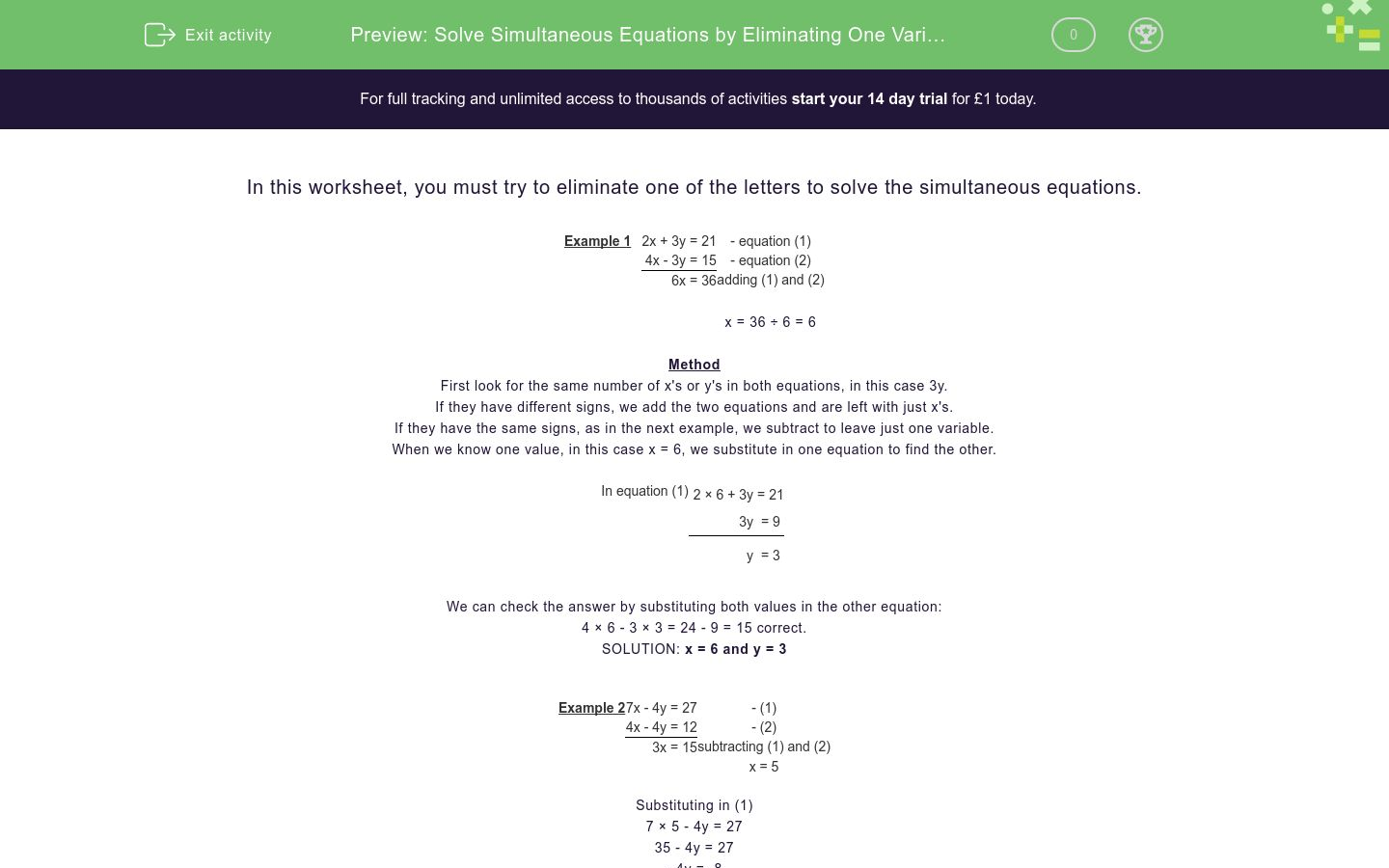# Solve Simultaneous Equations by Eliminating One Variable

In this worksheet, students solve simultaneous equations by eliminating one variable.Key stage:  KS 3

Curriculum topic:   Algebra

Curriculum subtopic:   Understand Expressions, Equations, Inequalities, Terms and Factors

Difficulty level:#### Worksheet Overview

In this worksheet, you must try to eliminate one of the letters to solve the simultaneous equations.

Example 1
 2x + 3y = 21 - equation (1) 4x - 3y = 15 - equation (2) 6x = 36 adding (1) and (2) x = 36 ÷ 6 = 6

Method

First look for the same number of x's or y's in both equations, in this case 3y.

If they have different signs, we add the two equations and are left with just x's.

If they have the same signs, as in the next example, we subtract to leave just one variable.

When we know one value, in this case x = 6, we substitute in one equation to find the other.

In equation (1)
 2 × 6 + 3y = 21 3y = 9 y = 3

We can check the answer by substituting both values in the other equation:

4 × 6 - 3 × 3 = 24 - 9 = 15 correct.

SOLUTION: x = 6 and y = 3

Example 2
 7x - 4y = 27 - (1) 4x - 4y = 12 - (2) 3x = 15 subtracting (1) and (2) x = 5

Substituting in (1)

7 × 5 - 4y = 27

35 - 4y = 27

- 4y = -8

y = 2

Checking in (2) 4 × 5 - 4 × 2 = 20 - 8 = 12 correct.

SOLUTION: x = 5 and y = 2

### What is EdPlace?

We're your National Curriculum aligned online education content provider helping each child succeed in English, maths and science from year 1 to GCSE. With an EdPlace account you’ll be able to track and measure progress, helping each child achieve their best. We build confidence and attainment by personalising each child’s learning at a level that suits them.

Get started